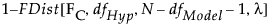Fitting Linear Models > Statistical Details > Power Calculations > Computations for the Adjusted Power
Publication date: 05/24/2021

## Computations for the Adjusted Power

The adjusted power calculation (Wright and O’Brien 1988) is relevant only for retrospective power analysis. Adjusted power calculates power using a noncentrality parameter estimate that has been adjusted to remove the positive bias that occurs when parameters are simply replaced by their sample estimates.

The estimate of the noncentrality parameter, λ, obtained by estimating δ and σ by their sample estimates, is calculated as follows: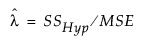Wright and O’Brien (1988) explain that an unbiased estimate of the noncentrality parameter is given by the following: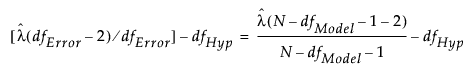The expression on the right illustrates the calculation of the unbiased noncentrality parameter when a sample size N, different from the study size n, is proposed for a retrospective power analysis. Here, dfHyp represents the degrees of freedom for the hypothesis and dfModel represents the degrees of freedom for the whole model.

Unfortunately, this adjustment to the noncentrality estimate can lead to negative values. Negative values are set to zero, reintroducing some slight bias. The adjusted noncentrality estimate is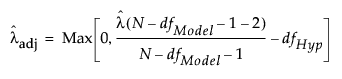Poweradj =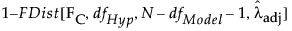Confidence limits for the noncentrality parameter are constructed as described in Dwass (1955):

Lower CL for λ =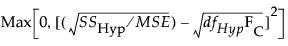Upper CL for λ =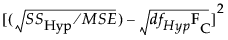Confidence limits for the power are obtained by substituting these confidence limits for λ into the following equation:

Power =